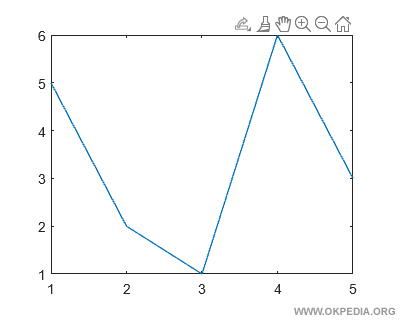# How to make 2D graph in Matlab and Octave

To make 2D graph of a function in Matlab and Octave, use the function plot(x,y)

plot(x,y)

• The first parameter (x) is an array with the x axis values
• The second parameter (y) is an array with the y-axis values

The two arrays make up the coordinates (x, y) of the points of the graph on the Cartesian diagram.

Note.The two arrays x and y must have the same number of elements.

## Examples

Example 1

Create an array with x-axis values

>> x = [ 1 2 3 4 5 ]

Create another array with y-axis values

>> y = [ 5 2 1 6 3 ]

Both x and y arrays have five elements.

Draw the graph of the function using plot(x,y) command

>> plot(x,y)

This command draws the graph of the function on the screen.Example 2

Create an array containing 100 elements

>> x = linspace(1,100);

Create another y array containing the natural log (x) values for each array x value.

>> y = log(x);

Both x and y arrays have one hundred elements.

To draw the graph of the function, type plot(x,y)

>> plot(x,y)

This command draws the graph of the natural logarithm from 1 to 100.https://how.okpedia.org/en/matlab/how-to-make-2d-graph-in-matlab-and-octaveReport us an error or send a suggestion to improve this page

Matlab and Octave